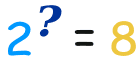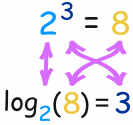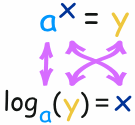# Logarithm

Welcome to class!

In today’s class, we will be talking about logarithm. Enjoy the class!

### LogarithmWhat is a logarithm?

Logarithms are another way of thinking about exponents.

Example: How many 2s do we multiply to get 8?

Answer: 2 × 2 × 2 = 8, so we had to multiply 3 of the 2s to get 8

So, the logarithm is 3

So, these two things are the same:

(2 x 2 x 2 = 8 )  ≡    ( log2(8) = 3)

#### How to write it

We write “the number of 2s we need to multiply to get 8 is 3” as:

log2(8) = 3

The number we multiply is called the “base”, so we can say:

• “the logarithm of 8 with base 2 is 3”
• or “log base 2 of 8 is 3”
• or “the base-2 log of 8 is 3”

Notice we are dealing with three numbers:

• the base: the number we are multiplying (a “2” in the example above)
• how often to use it in a multiplication (3 times, which is the logarithm)
• The number we want to get (an “8”)
##### Example: What is log5(625) …?

We are asking “how many 5s need to be multiplied together to get 625?”

5 × 5 × 5 × 5 = 625, so we need 4 of the 5s

##### Example: What is log2(64) …?

We are asking “how many 2s need to be multiplied together to get 64?”

2 × 2 × 2 × 2 × 2 × 2 = 64, so we need 6 of the 2s

#### Exponents

Exponents and Logarithms are related, let’s find out how …

 The exponent says how many times to use the number in a multiplication. In this example: 23 = 2 × 2 × 2 = 8 (2 is used 3 times in multiplication to get 8)

So a logarithm answers a question like this:In this way:The logarithm tells us what the exponent is!

In that example, the “base” is 2 and the “exponent” is 3:

So, the logarithm answers the question:

What exponent do we need?

(for one number to become another number) ?

The general case is:Example: What is log10(100) …?

102 = 100

So, an exponent of 2 is needed to make 10 into 100, and:

log10(100) = 2

Example: What is log3(81) …?

34 = 81

So, an exponent of 4 is needed to make 3 into 81, and:

log3(81) = 4

In our next class, we will be talking about the Quadratic Equation by Factorization and Completing the Square Method.  We hope you enjoyed the class.

Should you have any further question, feel free to ask in the comment section below and trust us to respond as soon as possible.

Everybody SCREAM💃 ClassNotes evolving to Afrilearn! Get ready for a world-class learning experience🎊

### 5 thoughts on “Logarithm”

1.Wow i really love this class and today is my first day

1.Welcome to ClassNotes.ng Annabel! We’re glad to have you here too!

2.Hi everyone I am enjoying this class it is my first day

3.Hi everyone

4.I’m really enjoying
And i’m also understanding it
I like the way they broke it down for me to understand better
And the explaination is amazing
It’s also my first day and i understand it so well
Keep it guys
Love this class

Don`t copy text!